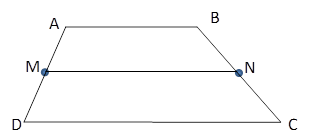Chapter 4.4, Problem 15E### Elementary Geometry for College St...

6th Edition
Daniel C. Alexander + 1 other
ISBN: 9781285195698

#### Solutions

Chapter
Section### Elementary Geometry for College St...

6th Edition
Daniel C. Alexander + 1 other
ISBN: 9781285195698
Textbook Problem
1 views

# In Exercises 11 to 16, the drawing shows trapezoid ABCD with A B ¯ | | D C ¯ ; also, M and N are midpoints of A D ¯ and B C ¯ , respectively.Exercises 11-16Given: A B = 6 x + 5 , D C = 8 x − 1 .Find: MN, in terms of x .

To determine

To Find:

MN in terms of x for the trapezoid ABCD.

Explanation

Consider the following trapezoid ABCD.

Given:

AB=6x+5, DC=8x1.

Also in the given trapezoid, AB¯||DC¯.

M is the midpoint of the side AD¯ and N is the midpoint of the side BC¯

### Still sussing out bartleby?

Check out a sample textbook solution.

See a sample solution

#### The Solution to Your Study Problems

Bartleby provides explanations to thousands of textbook problems written by our experts, many with advanced degrees!

Get Started

#### In Exercises 33-38, rewrite the expression using positive exponents only. 34. 3s1/3 s7/3

Applied Calculus for the Managerial, Life, and Social Sciences: A Brief Approach

#### In Problems 30-36, solve each system using matrices. 35.

Mathematical Applications for the Management, Life, and Social Sciences

#### If x = ln( sec + tan ), show that sec = cosh x.

Single Variable Calculus: Early Transcendentals, Volume I

#### In the graph at the right, limx1f(x)=. a) 2 b) 1 c) 3 d) does not exist

Study Guide for Stewart's Single Variable Calculus: Early Transcendentals, 8th